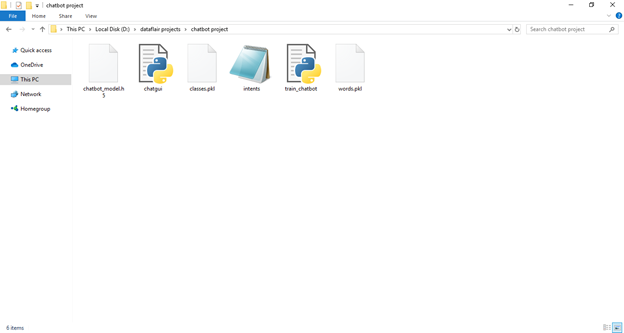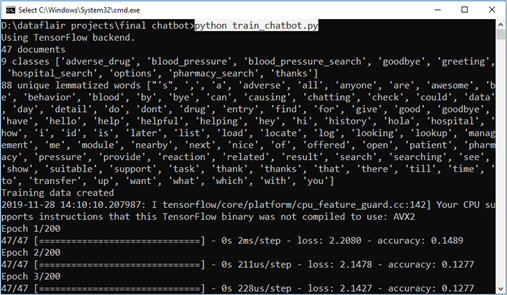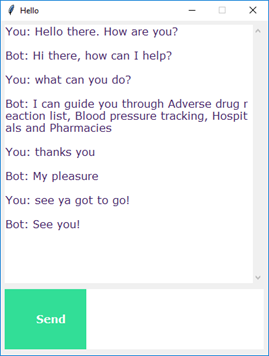### Learn to build your first chatbot using NLTK & Keras

In this Python project with source code, we are going to build a chatbot using deep learning
techniques. The chatbot will be trained on the dataset which contains categories (intents), pattern and responses. We use a special recurrent neural network (LSTM) to classify which category the users message belongs to and then we will give a random response from the list of responses.

Lets create a retrieval based chatbot using NLTK, Keras, Python, etc.

The Dataset

The dataset we will be using is intents.json. This is a JSON file that contains the patterns we need
to find and the responses we want to return to the user.

Prerequisites

The project requires you to have good knowledge of Python, Keras, and Natural language processing (NLTK). Along with them, we will use some helping modules which you can download using the python-pip command.

`pip install tensorflow, keras, pickle, nltk`

How to Make Chatbot in Python?

Now we are going to build the chatbot using Python but first, let us see the file structure and the
type of files we will be creating:*     Intents.json - The data file which has predefined patterns and responses.

*     train_chatbot.py - In this Python file, we wrote a script to build the model and train our chatbot.

*     Words.pkl - This is a pickle file in which we store the words Python object that contains a list of our vocabulary.

*     Classes.pkl - The classes pickle file contains the list of categories.

*     Chatbot_model.h5 - This is the trained model that contains information about the model and has weights of the neurons.

*     Chatgui.py - This is the Python script in which we implemented GUI for our chatbot. Users can easily interact with the bot.

Here are the 5 steps to create a chatbot in Python from scratch:

1. Import and load the data file

2. Preprocess data

3.   Create training and testing data

4.   Build the model

5.   Predict the response

1. Import and load the data file

First, make a file name as train_chatbot.py. We import the necessary packages for our chatbot and
initialize the variables we will use in our Python project.

The data file is in JSON format so we used the json package to parse the JSON file into Python.

`import nltkfrom nltk.stem import WordNetLemmatizerlemmatizer = WordNetLemmatizer()import jsonimport pickleimport numpy as npfrom keras.models import Sequentialfrom keras.layers import Dense, Activation, Dropoutfrom keras.optimizers import SGDimport randomwords=[]classes = []documents = []ignore_words = ['?', '!']data_file = open('intents.json').read()intents = json.loads(data_file)`

2. Preprocess data

When working with text data, we need to perform various preprocessing on the data before we make a
machine learning or a deep learning model. Tokenizing is the most basic and first thing you can do on text data. Tokenizing is the process of breaking the whole text into small parts like words.

Here we iterate through the patterns and tokenize the sentence using nltk.word_tokenize() function and
append each word in the words list. We also create a list of classes for our tags.

`for intent in intents['intents']:for pattern in intent['patterns']:#tokenize each wordw = nltk.word_tokenize(pattern)words.extend(w)#add documents in the corpusdocuments.append((w, intent['tag']))# add to our classes listif intent['tag'] not in classes:classes.append(intent['tag'])`

Now we will lemmatize each word and remove duplicate words from the list. Lemmatizing is the process of converting a word into its lemma form and then creating a pickle file to store the Python objects which we will use while predicting.

`# lemmatize, lower each word and remove duplicateswords = [lemmatizer.lemmatize(w.lower()) for w in words if w not in ignore_words]words = sorted(list(set(words)))# sort classesclasses = sorted(list(set(classes)))# documents = combination between patterns and intentsprint (len(documents), "documents")# classes = intentsprint (len(classes), "classes", classes)# words = all words, vocabularyprint (len(words), "unique lemmatized words", words)pickle.dump(words,open('words.pkl','wb'))pickle.dump(classes,open('classes.pkl','wb'))`

3. Create training and testing data

Now, we will create the training data in which we will provide the input and the output. Our input will
be the pattern and output will be the class our input pattern belongs to. But the computer doesnt understand text so we will convert text into numbers.

`# create our training datatraining = []# create an empty array for our outputoutput_empty =  * len(classes)# training set, bag of words for each sentencefor doc in documents:# initialize our bag of wordsbag = []# list of tokenized words for the patternpattern_words = doc# lemmatize each word - create base word, in attempt to represent related wordspattern_words = [lemmatizer.lemmatize(word.lower()) for word in pattern_words]# create our bag of words array with 1, if word match found in current patternfor w in words:bag.append(1) if w in pattern_words else bag.append(0)# output is a '0' for each tag and '1' for current tag (for each pattern)output_row = list(output_empty)output_row[classes.index(doc)] = 1training.append([bag, output_row])# shuffle our features and turn into np.arrayrandom.shuffle(training)training = np.array(training)# create train and test lists. X - patterns, Y - intentstrain_x = list(training[:,0])train_y = list(training[:,1])print("Training data created")`

4. Build the model

We have our training data ready, now we will build a deep neural network that has 3 layers. We use
the Keras sequential API for this. After training the model for 200 epochs, we achieved 100% accuracy on our model. Let us save the model as chatbot_model.h5.

`# Create model - 3 layers. First layer 128 neurons, second layer 64 neurons and 3rd output layer contains number of neurons# equal to number of intents to predict output intent with softmaxmodel = Sequential()model.add(Dense(128, input_shape=(len(train_x),), activation='relu'))model.add(Dropout(0.5))model.add(Dense(64, activation='relu'))model.add(Dropout(0.5))model.add(Dense(len(train_y), activation='softmax'))# Compile model. Stochastic gradient descent with Nesterov accelerated gradient gives good results for this modelsgd = SGD(lr=0.01, decay=1e-6, momentum=0.9, nesterov=True)model.compile(loss='categorical_crossentropy', optimizer=sgd, metrics=['accuracy'])#fitting and saving the model hist = model.fit(np.array(train_x), np.array(train_y), epochs=200, batch_size=5, verbose=1)model.save('chatbot_model.h5', hist)print("model created")`

5. Predict the response (Graphical User Interface)

Now to predict the sentences and get a response from the user to let us create a new file chatapp.py.

We will load the trained model and then use a graphical user interface that will predict the response from the bot. The model will only tell us the class it belongs to, so we will implement some functions which will identify the class and then retrieve us a random response from the list of responses.

Again we import the necessary packages and load the words.pkl and classes.pkl pickle files which we have created when we trained our model:

`import nltkfrom nltk.stem import WordNetLemmatizerlemmatizer = WordNetLemmatizer()import pickleimport numpy as npfrom keras.models import load_modelmodel = load_model('chatbot_model.h5')import jsonimport randomintents = json.loads(open('intents.json').read())words = pickle.load(open('words.pkl','rb'))classes = pickle.load(open('classes.pkl','rb'))`

To predict the class, we will need to provide input in the same way as we did while training. So we will create some functions that will perform text preprocessing and then predict the class.

`def clean_up_sentence(sentence):# tokenize the pattern - split words into arraysentence_words = nltk.word_tokenize(sentence)# stem each word - create short form for wordsentence_words = [lemmatizer.lemmatize(word.lower()) for word in sentence_words]return sentence_words# return bag of words array: 0 or 1 for each word in the bag that exists in the sentencedef bow(sentence, words, show_details=True):# tokenize the patternsentence_words = clean_up_sentence(sentence)# bag of words - matrix of N words, vocabulary matrixbag = *len(words) for s in sentence_words:for i,w in enumerate(words):if w == s: # assign 1 if current word is in the vocabulary positionbag[i] = 1if show_details:print ("found in bag: %s" % w)return(np.array(bag))def predict_class(sentence, model):# filter out predictions below a thresholdp = bow(sentence, words,show_details=False)res = model.predict(np.array([p]))ERROR_THRESHOLD = 0.25results = [[i,r] for i,r in enumerate(res) if r>ERROR_THRESHOLD]# sort by strength of probabilityresults.sort(key=lambda x: x, reverse=True)return_list = []for r in results:return_list.append({"intent": classes[r], "probability": str(r)})return return_list`

After predicting the class, we will get a random response from the list of intents.

`def getResponse(ints, intents_json):tag = ints['intent']list_of_intents = intents_json['intents']for i in list_of_intents:if(i['tag']== tag):result = random.choice(i['responses'])breakreturn resultdef chatbot_response(text):ints = predict_class(text, model)res = getResponse(ints, intents)return res`

Now we will code a graphical user interface. For this, we use the Tkinter library which already comes in python. We will take the input message from the user and then use the helper functions we have created to get the response from the bot and display it on the GUI. Here is the full source code for the GUI.

`#Creating GUI with tkinterimport tkinterfrom tkinter import *def send():msg = EntryBox.get("1.0",'end-1c').strip()EntryBox.delete("0.0",END)if msg != '':ChatLog.config(state=NORMAL)ChatLog.insert(END, "You: " + msg + '\n\n')ChatLog.config(foreground="#442265", font=("Verdana", 12 ))res = chatbot_response(msg)ChatLog.insert(END, "Bot: " + res + '\n\n')ChatLog.config(state=DISABLED)ChatLog.yview(END)base = Tk()base.title("Hello")base.geometry("400x500")base.resizable(width=FALSE, height=FALSE)#Create Chat windowChatLog = Text(base, bd=0, bg="white", height="8", width="50", font="Arial",)ChatLog.config(state=DISABLED)#Bind scrollbar to Chat windowscrollbar = Scrollbar(base, command=ChatLog.yview, cursor="heart")ChatLog['yscrollcommand'] = scrollbar.set#Create Button to send messageSendButton = Button(base, font=("Verdana",12,'bold'), text="Send", width="12", height=5,bd=0, bg="#32de97", activebackground="#3c9d9b",fg='#ffffff',command= send )#Create the box to enter messageEntryBox = Text(base, bd=0, bg="white",width="29", height="5", font="Arial")#EntryBox.bind("<Return>", send)#Place all components on the screenscrollbar.place(x=376,y=6, height=386)ChatLog.place(x=6,y=6, height=386, width=370)EntryBox.place(x=128, y=401, height=90, width=265)SendButton.place(x=6, y=401, height=90)base.mainloop()`

6. Run the chatbot

To run the chatbot, we have two main files; train_chatbot.py and chatapp.py.

First, we train the model using the command in the terminal:

`python train_chatbot.py`

If we dont see any error during training, we have successfully created the model. Then to run the
app, we run the second file.

`python chatgui.py`

The program will open up a GUI window within a few seconds. With the GUI you can easily chat with the bot.

Output:---------------------------------------------

End.

Please comment below any questions or article requests.
Like the articles and Follow me to get notified when I post another article.

Cheers!#### More Articles of Rishabh Karmakar:

Name Views Likes
Basic Date and Time in Python 165 1
Using Defaultdict in Python 55 1
Floor Division in Python 204 1
Python time sleep function 77 1
Immutable Objects in Python 78 1
Mutable Objects in Python 81 1
Python Escape Sequences 70 1
Escape Sequence in Python 0 0
Python Bytearray 65 1
Plotting Histogram in Python 138 1
Using Voluptuous in Python 173 1
TRAINING MACHINE LEARNING MODEL WITH TENSORFLOW CLOUD 257 1
Pandas - ModuleNotFoundError - No Module Named Pandas and Functions Explained 5477 5
OpenCV - ModuleNotFoundError - No Module Named cv2 and Algorithms Explained 344 5
OpenCV - ModuleNotFoundError - No Module Named 274 9
Simple Python Project Mario Shooter Game 388 4
Web crawling using Python and Scrapy 266 6
CNN vs ANN vs RNN 294 6
Deep Learning - Activation and Loss Function 249 6
CNN vs. RNN vs. ANN 42 10
Advanced Python Projects Predicting and Forecasting Stock Market Prices 562 6
Intermediate Python Projects Tensorflow Live Object Detection 329 7
Intermediate Python Projects Optical Character and Text Recognizer from Live Images 336 7
Advanced Python Projects Analysing Music Trends and Recommendations 468 7
Advanced Python Projects Python Chatbot using NLTK and Keras 760 7
Advanced Python Projects CIFAR-10 Image Classification using Deep Learning 554 9
Advanced Python Projects Image Caption Generator using CNN and LSTM 454 10
Advanced Python Project Breast Cancer Classification using SVR 373 10
Advanced Python Project Smiling Face Detector using CNN 445 10
Advanced Python Project Handwritten Digit Recognizer 506 11
Intermediate Python Project Speed Typing in Python 456 10
Advanced Python Project Next Alphabet or Word Prediction using LSTM 365 12
Intermediate Python Project Find most similar word using Word2Vec 288 10
Advanced Python Projects IMDB Movie Review Sentiment Analysis 261 10
Intermediate Python Project Detection of Real or Fake News 282 10
Advanced Python Project Credit Card Fraud Detection 264 10
Intermediate Python Project Snake Game Pygame 326 10
Simple Python Projects Make a multi-colored rainbow pattern in Python 400 10
Advanced Project Analysis with Sentiment Classification using Bidirectional Recurrent Neural Network 244 10
Advanced Project Forest Fire Prediction using SVR Random Forest and Deep NN 380 10
Advanced Project Sentiment and WordCloud Analysis of Online Reviews 337 12
Python - Synthetic Data Generator for Machine Learning and Artificial Intelligence 263 9
Advanced Project Deep Dream AI 269 10
Python - Symbolic regression classification generator 271 9
Generate Polynomial Functions and Random Function Generator - Python 286 10
Intermediate Project Sentiment and WordCloud Analysis of Women E-Commerce 287 10
Intermediate Project Iris Data Classification 226 10
Intermediate Project Titanic Classification using Decision Tree 248 10
Machine Learning - Decision Tree in Python 296 9
Python - All about lambda functions 251 9
Machine Learning - Polynomial Regression in Python 278 9
Machine Learning - Multiple Linear Regression in Python 260 11
Machine Learning - Simple Linear Regression in Python 244 9
Implement all 2D and 3D types plots in Python 236 9
Implement 1D, 2D and 3D CNN in Python 310 9
Simple Python Projects Multiplayer Tic-Tac-Toe 273 11
Problem - Validating Credit Card Numbers Hackerrank 275 9
Problem - Linear Algebra using Numpy Hackerrank 712 9
Problem - Basic Spell Checker Hackerrank 214 9
Implement Binary Tree in Python 277 9
Implement Graph in Python 262 9
Python for Data Science 213 9
Simple Python Projects Select Region of Interest - OpenCV 324 10
Simple Python Projects Code to mask white pixels in a coloured image - OpenCV 336 10
Simple Python Projects Code to mask white pixels in a gray scale image - OpenCV 297 10
Simple Python Projects Convert colour image to gray scale and apply cartoon effects - OpenCV 347 10
Advanced Project - Automatic Facial Recognition Based Attendance System 717 9
Simple Python Projects Live Talking Counting Clock - Pyttsx3, Tkinter 263 10
Python Simple and Fast Text to Speech 228 9
Simple Python Projects Simple Zodiac Sign Teller 233 10
Simple Python Projects Simple Fortune Teller Game 275 10
Simple Python Projects Simple Guessing Game 390 10
Implementing Multi-line Strings 202 9
Save and Load Machine Learning Models in Python with scikit-learn 399 9
Use of Ternary operator for conditional assignment. 350 9
Chaining comparison operators 215 9
Implement Circular Singly Linked List 241 9
Implementing Command Line Arguments 356 9
Solve Knapsack Problem Using Dynamic Programming 386 10